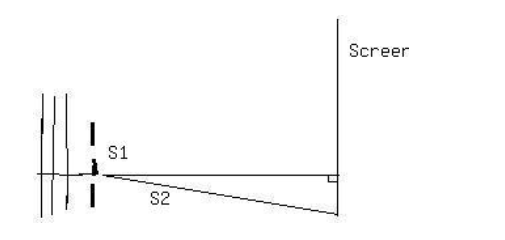Ask question

# The sum of two acute angles is an acute angle. always true, never or sometimes. Explain.# The sum of two acute angles is an acute angle. always true, never or sometimes. Explain.

Question
Right triangles and trigonometryasked 2021-01-02
The sum of two acute angles is an acute angle. always true, never or sometimes. Explain.

## Answers (1)2021-01-03
Sometimes true
Recall that an acute angle measures greater than 0°0° and less than 90°.
An example where the statement is true is when the two angles are 10° and 20° which sums to 30°, an acute angle.
An example where the statement is false is when the two angles are 60°60° and 70°70° which sums to 130°, an obtuse angle.

### Relevant Questionsasked 2021-03-12
Aidan knows that the observation deck on the Vancouver Lookout is 130 m above the ground. He measures the angle between the ground and his line of sight to the observation deck as $$\displaystyle{77}^{\circ}$$. How far is Aidan from the base of the Lookout to the nearest metre?asked 2020-10-18
Use function notation to describe the way the second variable (DV) depends upon the first variable (IV). Determine the domain and range for each, determine if there is a positive, negative, or no relationship, and explain your answers.
A)IV: an acute angle V in a right triangle: DV: the area B of the triangle if the hypotenuse is a fixed length G.
B)IV: one leg P of a right triangle: DV: the hypotenuse G of the right triangle if the other leg is 2
C)IV: the hypotenuse G of a right triangle: DV: the other leg P of the right triangle is one leg is 5.asked 2021-02-08
Determine whether each statement is sometimes, always , or never true for matrices A and B and explain your reasoning. Stuck on one of them? Try a few examples.
a) If A+B exists , then A-B exists
b) If A and B have the same number of elements , then A+B exists.asked 2020-12-27
Given theta is an acute angle such that sin theta = $$\displaystyle\frac{{5}}{{13}}$$ find the value of tan (theta - pi\4)asked 2021-03-30
A spring gun projects a golf ball at an angle of 45 degreesabove the horizontal. The ball has horizontal range of 32 feet.what is the maxium height to which the ball can rise? and what are2 angles for the range is 20 ft.asked 2021-05-181. S1 and S2, shown above, are thin parallel slits in an opaqueplate. A plane wave of wavelength λ is incident from the leftmoving in a direction perpendicular to the plate. On a screenfar from the slits there are maximums and minimums in intensity atvarious angles measured from the center line. As the angle isincreased from zero, the first minimum occurs at 3 degrees. Thenext minimum occurs at an angle of-
A. 4.5 degrees
B. 6 degrees
C. 7.5 degrees
D. 9 degrees
E. 12 degreesasked 2021-01-15
The converse of the Pythagorean theorem is also a true statement: If the sum of the squares of the lengths of two sides of a triangle is equal to the square of the length of the third side, then the triangle is a right triangle. Use the distance formula and the Pythagorean theorem to determine whether the set of points could be vertices of a right triangle.
(-4,3), (0,5), and (3,-4)asked 2021-02-03
The converse of the Pythagorean theorem is also a true statement: If the sum of the squares of the lengths of two sides of a triangle is equal to the square of the length of the third side, then the triangle is a right triangle. Use the distance formula and the Pythagorean theorem to determine whether the set of points could be vertices of a right triangle.
(-3,1), (2,-1), and (6,9)asked 2020-12-29
The converse of the Pythagorean theorem is also a true statement: If the sum of the squares of the lengths of two sides of a triangle is equal to the square of the length of the third side, then the triangle is a right triangle. Use the distance formula and the Pythagorean theorem to determine whether the set of points could be vertices of a right triangle.
(-3,1), (2,-1), and (6,9)asked 2021-02-02
The converse of the Pythagorean theorem is also a true statement: If the sum of the squares of the lengths of two sides of a triangle is equal to the square of the length of the third side, then the triangle is a right triangle. Use the distance formula and the Pythagorean theorem to determine whether the set of points could be vertices of a right triangle.
(-3,1), (2,-1), and (6,9)
...